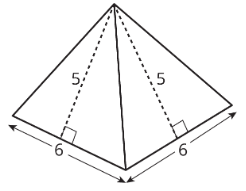$$\newcommand{\id}{\mathrm{id}}$$ $$\newcommand{\Span}{\mathrm{span}}$$ $$\newcommand{\kernel}{\mathrm{null}\,}$$ $$\newcommand{\range}{\mathrm{range}\,}$$ $$\newcommand{\RealPart}{\mathrm{Re}}$$ $$\newcommand{\ImaginaryPart}{\mathrm{Im}}$$ $$\newcommand{\Argument}{\mathrm{Arg}}$$ $$\newcommand{\norm}{\| #1 \|}$$ $$\newcommand{\inner}{\langle #1, #2 \rangle}$$ $$\newcommand{\Span}{\mathrm{span}}$$

# 1.5.4: More Nets, More Surface Area

•• Contributed by Illustrative Mathematics
• OpenUp Resources
$$\newcommand{\vecs}{\overset { \rightharpoonup} {\mathbf{#1}} }$$ $$\newcommand{\vecd}{\overset{-\!-\!\rightharpoonup}{\vphantom{a}\smash {#1}}}$$$$\newcommand{\id}{\mathrm{id}}$$ $$\newcommand{\Span}{\mathrm{span}}$$ $$\newcommand{\kernel}{\mathrm{null}\,}$$ $$\newcommand{\range}{\mathrm{range}\,}$$ $$\newcommand{\RealPart}{\mathrm{Re}}$$ $$\newcommand{\ImaginaryPart}{\mathrm{Im}}$$ $$\newcommand{\Argument}{\mathrm{Arg}}$$ $$\newcommand{\norm}{\| #1 \|}$$ $$\newcommand{\inner}{\langle #1, #2 \rangle}$$ $$\newcommand{\Span}{\mathrm{span}}$$ $$\newcommand{\id}{\mathrm{id}}$$ $$\newcommand{\Span}{\mathrm{span}}$$ $$\newcommand{\kernel}{\mathrm{null}\,}$$ $$\newcommand{\range}{\mathrm{range}\,}$$ $$\newcommand{\RealPart}{\mathrm{Re}}$$ $$\newcommand{\ImaginaryPart}{\mathrm{Im}}$$ $$\newcommand{\Argument}{\mathrm{Arg}}$$ $$\newcommand{\norm}{\| #1 \|}$$ $$\newcommand{\inner}{\langle #1, #2 \rangle}$$ $$\newcommand{\Span}{\mathrm{span}}$$

## Lesson

Let's draw nets and find the surface area of polyhedra.

Exercise $$\PageIndex{1}$$: Notice and Wonder: Wrapping Paper

Kiran is wrapping this box of sports cards as a present for a friend.Figure $$\PageIndex{1}$$

What do you notice? What do you wonder?

Exercise $$\PageIndex{2}$$: Building Prisms and Pyramids

Your teacher will give you a drawing of a polyhedron. You will draw its net and calculate its surface area.

1. What polyhedron do you have?
2. Study your polyhedron. Then, draw its net on graph paper. Use the side length of a grid square as the unit.
3. Label each polygon on the net with a name or number.
4. Find the surface area of your polyhedron. Show your thinking in an organized manner so that it can be followed by others.

Exercise $$\PageIndex{3}$$: Comparing Boxes

Here are the nets of three cardboard boxes that are all rectangular prisms. The boxes will be packed with 1-centimeter cubes. All lengths are in centimeters.Figure $$\PageIndex{2}$$
1. Compare the surface areas of the boxes. Which box will use the least cardboard? Show your reasoning.
2. Now compare the volumes of these boxes in cubic centimeters. Which box will hold the most 1-centimeter cubes? Show your reasoning.

Figure C shows a net of a cube. Draw a different net of a cube. Draw another one. And then another one. How many different nets can be drawn and assembled into a cube?

### Summary

The surface area of a polyhedron is the sum of the areas of all of the faces. Because a net shows us all faces of a polyhedron at once, it can help us find the surface area. We can find the areas of all polygons in the net and add them.Figure $$\PageIndex{3}$$Figure $$\PageIndex{4}$$

A square pyramid has a square and four triangles for its faces. Its surface area is the sum of the areas of the square base and the four triangular faces:

$$(6\cdot 6)+4\cdot (\frac{1}{2}\cdot 5\cdot 6)=96$$

The surface area of this square pyramid is 96 square units.

### Glossary Entries

Definition: Base (of a Prism or Pyramid)

The word base can also refer to a face of a polyhedron.

A prism has two identical bases that are parallel. A pyramid has one base.

A prism or pyramid is named for the shape of its base.Figure $$\PageIndex{5}$$: The figure on the left is labeled pentagonal prism. There are two identical pentagons on the top and bottom. Each vertex of a pentagon is connected by a vertical segment to the corresponding vertex of the other pentagons. The pentagons are each shaded, with the word base pointing to each. The figure on the right is labeled hexagonal pyramid. There is a hexagon on the bottom shaded green. From a point above the hexagon extend 6 segments, each connected to a vertex of the hexagon.

Definition: Face

Each flat side of a polyhedron is called a face. For example, a cube has 6 faces, and they are all squares.

Definition: Net

A net is a two-dimensional figure that can be folded to make a polyhedron.

Here is a net for a cube.Figure $$\PageIndex{6}$$

Definition: Polyhedron

A polyhedron is a closed, three-dimensional shape with flat sides. When we have more than one polyhedron, we call them polyhedra.

Here are some drawings of polyhedra.Figure $$\PageIndex{7}$$

Definition: Prism

A prism is a type of polyhedron that has two bases that are identical copies of each other. The bases are connected by rectangles or parallelograms.

Here are some drawings of prisms.Figure $$\PageIndex{8}$$

Definition: Pyramid

A pyramid is a type of polyhedron that has one base. All the other faces are triangles, and they all meet at a single vertex.

Here are some drawings of pyramids.Figure $$\PageIndex{9}$$

Definition: Surface Area

The surface area of a polyhedron is the number of square units that covers all the faces of the polyhedron, without any gaps or overlaps.

For example, if the faces of a cube each have an area of 9 cm2, then the surface area of the cube is $$6\cdot 9$$, or 54 cm2.

## Practice

Exercise $$\PageIndex{4}$$

Jada drew a net for a polyhedron and calculated its surface area.Figure $$\PageIndex{10}$$: Triangular prism, Faces are: 5 by 4 rectangle, area 20 square centimeters. 4 by 4 square, area 16 square centimeters, 2 triangles, bases of 5, heights of 3, areas of 12 square centimeters each, and 3 by 4 rectangle, area =12 square centimeters.
1. What polyhedron can be assembled from this net?
2. Jada made some mistakes in her area calculation. What were the mistakes?
3. Find the surface area of the polyhedron. Show your reasoning.

Exercise $$\PageIndex{5}$$

A cereal box is 8 inches by 2 inches by 12 inches. What is its surface area? Show your reasoning. If you get stuck, consider drawing a sketch of the box or its net and labeling the edges with their measurements.

Exercise $$\PageIndex{6}$$

Twelve cubes are stacked to make this figure.Figure $$\PageIndex{11}$$
1. What is its surface area?
2. How would the surface area change if the top two cubes are removed?

(From Unit 1.5.1)

Exercise $$\PageIndex{7}$$

Here are two polyhedra and their nets. Label all edges in the net with the correct lengths.Figure $$\PageIndex{12}$$Figure $$\PageIndex{13}$$

Exercise $$\PageIndex{8}$$

1. What three-dimensional figure can be assembled from the net?Figure $$\PageIndex{14}$$
1. What is the surface area of the figure? (One grid square is 1 square unit.)

(From Unit 1.5.3)